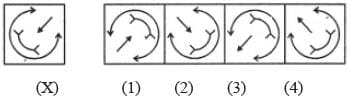# Olympiad Test: Mirror Images -1

Test Description

## 20 Questions MCQ Test Science Olympiad Class 7 | Olympiad Test: Mirror Images -1

Olympiad Test: Mirror Images -1 for Class 7 2023 is part of Science Olympiad Class 7 preparation. The Olympiad Test: Mirror Images -1 questions and answers have been prepared according to the Class 7 exam syllabus.The Olympiad Test: Mirror Images -1 MCQs are made for Class 7 2023 Exam. Find important definitions, questions, notes, meanings, examples, exercises, MCQs and online tests for Olympiad Test: Mirror Images -1 below.
 1 Crore+ students have signed up on EduRev. Have you?
Olympiad Test: Mirror Images -1 - Question 1

### Directions: In each of the following questions you are given a combination of alphabets and/or numbers followed by four alternatives (1), (2), (3) and (4).  Q. Choose the alternative which closely resembles the mirror-image of the given combination.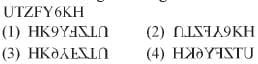Olympiad Test: Mirror Images -1 - Question 2

### Directions: In each of the following questions you are given a combination of alphabets and/or numbers followed by four alternatives (1), (2), (3) and (4).  Q. Choose the alternative which closely resembles the mirror-image of the given combination.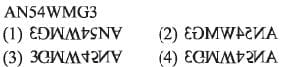Olympiad Test: Mirror Images -1 - Question 3

### Directions: In each of the following questions you are given a combination of alphabets and/or numbers followed by four alternatives (1), (2), (3) and (4).  Q. Choose the alternative which closely resembles the mirror-image of the given combination.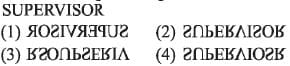Olympiad Test: Mirror Images -1 - Question 4

Directions: In each of the following questions you are given a combination of alphabets and/or numbers followed by four alternatives (1), (2), (3) and (4).

Q. Choose the alternative which closely resembles the mirror-image of the given combination.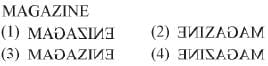Olympiad Test: Mirror Images -1 - Question 5

Directions: In each of the following questions you are given a combination of alphabets and/or numbers followed by four alternatives (1), (2), (3) and (4).

Q. Choose the alternative which closely resembles the mirror-image of the given combination.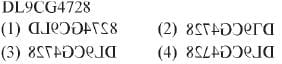Olympiad Test: Mirror Images -1 - Question 6

Directions: In each of the following questions you are given a combination of alphabets and/or numbers followed by four alternatives (1), (2), (3) and (4).

Q. Choose the alternative which closely resembles the mirror-image of the given combination.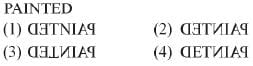Olympiad Test: Mirror Images -1 - Question 7

Directions: In each of the following questions you are given a combination of alphabets and/or numbers followed by four alternatives (1), (2), (3) and (4).

Q. Choose the alternative which closely resembles the mirror-image of the given combination.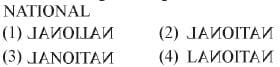Olympiad Test: Mirror Images -1 - Question 8

Directions: In each of the following questions you are given a combination of alphabets and/or numbers followed by four alternatives (1), (2), (3) and (4).

Q. Choose the alternative which closely resembles the mirror-image of the given combination.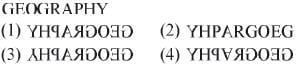Olympiad Test: Mirror Images -1 - Question 9

Directions: In each of the following questions you are given a combination of alphabets and/or numbers followed by four alternatives (1), (2), (3) and (4).

Q. Choose the alternative which closely resembles the mirror-image of the given combination.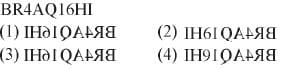Olympiad Test: Mirror Images -1 - Question 10

Directions: In each of the following questions you are given a combination of alphabets and/or numbers followed by four alternatives (1), (2), (3) and (4).

Q. Choose the alternative which closely resembles the mirror-image of the given combination.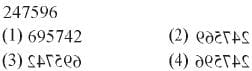Olympiad Test: Mirror Images -1 - Question 11

Directions: In each of the following questions you are given a combination of alphabets and/or numbers followed by four alternatives (1), (2), (3) and (4).

Q. Choose the alternative which closely resembles the mirror-image of the given combination.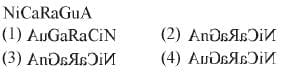Olympiad Test: Mirror Images -1 - Question 12

Directions: In each of the following questions you are given a combination of alphabets and/or numbers followed by four alternatives (1), (2), (3) and (4).

Q. Choose the alternative which closely resembles the mirror-image of the given combination.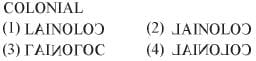Olympiad Test: Mirror Images -1 - Question 13

Directions: In each of the following questions you are given a combination of alphabets and/or numbers followed by four alternatives (1), (2), (3) and (4).

Q. Choose the alternative which closely resembles the mirror-image of the given combination.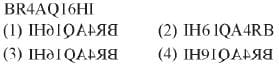Olympiad Test: Mirror Images -1 - Question 14

Directions: In each of the following questions you are given a combination of alphabets and/or numbers followed by four alternatives (1), (2), (3) and (4).

Q. Choose the alternative which closely resembles the mirror-image of the given combination.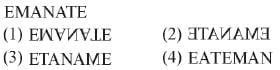Olympiad Test: Mirror Images -1 - Question 15

Directions: In each of the following questions you are given a combination of alphabets and/or numbers followed by four alternatives (1), (2), (3) and (4).

Q. Choose the alternative which closely resembles the mirror-image of the given combination.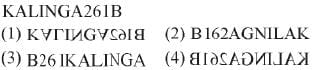Olympiad Test: Mirror Images -1 - Question 16

Directions: In each of the following questions, choose the correct mirror image of the given image of the Fig.(X) from amongst the four alternatives (1), (2), (3) and (4) given along with it.

Q. Choose the correct mirror image of the given figure (X) from amongst the four alternatives.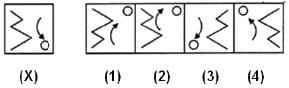Olympiad Test: Mirror Images -1 - Question 17

Directions: In each of the following questions, choose the correct mirror image of the given image of the Fig.(X) from amongst the four alternatives (1), (2), (3) and (4) given along with it.

Q. Choose the correct mirror image of the given figure (X) from amongst the four alternatives.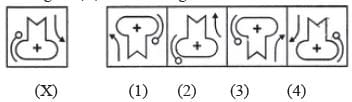Olympiad Test: Mirror Images -1 - Question 18

Directions: In each of the following questions, choose the correct mirror image of the given image of the Fig.(X) from amongst the four alternatives (1), (2), (3) and (4) given along with it.

Q. Choose the correct mirror image of the given figure (X) from amongst the four alternatives.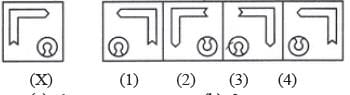Olympiad Test: Mirror Images -1 - Question 19

Directions: In each of the following questions, choose the correct mirror image of the given image of the Fig.(X) from amongst the four alternatives (1), (2), (3) and (4) given along with it.

Q. Choose the correct mirror image of the given figure (X) from amongst the four alternatives.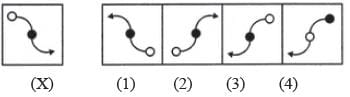Olympiad Test: Mirror Images -1 - Question 20

Directions: In each of the following questions, choose the correct mirror image of the given image of the Fig.(X) from amongst the four alternatives (1), (2), (3) and (4) given along with it.

Q. Choose the correct mirror image of the given figure (X) from amongst the four alternatives.